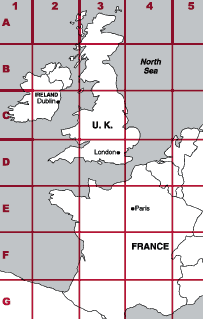# Coordinates

In mathematics, coordinates are a set of numbers that specify the position of a point in a coordinate system.

A number line is a 1D (one dimensional) coordinate system. A real number that matches the location of a point along a number line is called a coordinate of the point.

For the number line above, point A has a coordinate of -5, point B has a coordinate of 1, and the coordinate for point C is 4. Each coordinate tells you how far the point is to the left (if it is negative) or to the right (if it is positive) of the origin.

Many different coordinate systems exist. The most commonly used are the 2D (two dimensional) and 3D (three dimensional) coordinate systems.

## 2D coordinates

A 2D coordinate plane is formed by the intersection of a horizontal number line called the x-axis and a vertical number line called the y-axis. The two axes (plural for axis) intersect at a point called the origin.

### x- and y-coordinates

The x- and y-coordinates indicate the position of a point in the 2D coordinate plane relative to the origin. The coordinates are written as an ordered pair of numbers (x, y), where x indicates horizontal position and y indicates vertical position.

Example

The position of the point in the figure below is specified by the ordered pair, (6, 4). 6 is the x-coordinate and 4 is the y-coordinate of the point.

## 3D coordinates

A 3D coordinate system can be used to specify the position of a point in 3D space. A 3D coordinate plane is formed by the intersection of three axes that are perpendicular to each other at the origin. The three axes are labeled the x-axis, y-axis, and the z-axis.

The x-, y-, and z-axes can be drawn in a number of ways. The z-axis can be visualized as an extension of the 2D coordinate plane. Given that the x- and y- axes are the horizontal and vertical axes on a page, the z-axis can be visualized as coming out of, and going through the page, creating the third dimension. Since we cannot really draw a line coming out of a page, the 3D coordinate plane is more typically drawn as shown in the figure below, and how the axes are labeled can vary.

The position of a point in the 3D coordinate plane is specified similarly to that of a point in the 2D coordinate plane, with an added z-coordinate: (x, y, z).

Example

Points A and B are located at (7, 3, 5) and (-2, -7, 10) respectively. For each point, the first coordinate in the ordered triple is the x-coordinate, the second coordinate is the y-coordinate, and the third coordinate is the z-coordinate. To determine the location of each point in the 3D coordinate plane, count the specified number in the ordered triple for each coordinate, paying attention to whether or not to count in the positive or negative direction along the axes, as shown in the figure below.

### Did you know?

Coordinates are also used in maps. Often, maps will use a letter as one of the coordinates for locating a point, and will use a numeral for the other point. Coordinates for the map below include:Dublin, Ireland: C 2 London, England: D 3 Paris, France: E 4